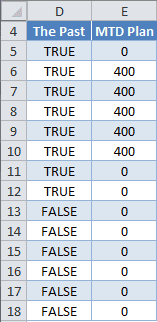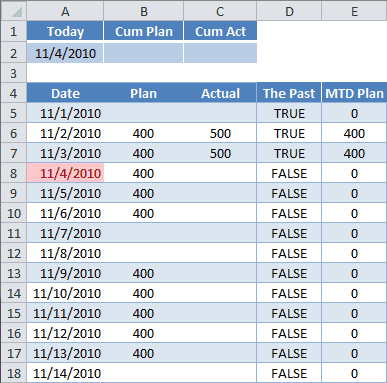# Single-Cell Array Formula in Excel

A Single-cell Array formula is powerful, yet can be hard to understand. The Evaluate Formula dialog box helps by revealing array values Excel holds in internal memory.

In the spreadsheet you see pictured, a single-cell array formula for the Cumulative Plan is located in cell B2. It summarizes the historical and current day’s Plan data. The Cumulative Actual formula, in cell C2, summarizes the Actual data in the same manner.

As an introduction to Array formulas, in my last post I added two columns. The multi-cell array formulas for columns D and E are:

{=\$A\$2>=A5:A18}

{=D5:D18*B5:B18}Both formulas are … Read the rest

# Multi-Cell Array Formula in Excel

Arrays are difficult to understand because Excel holds them internally and it’s hard to conceptualize how operations are performed on the data. I had a vexing problem that was solved by using a single-cell array formula and wanted to share it with you.

However, because of the subject nature of Arrays, I’m going to show the solution to this problem in two parts. Here I’ll cover a Multi-Cell Array formula and my next post will talk about a Single-Cell Array formula, which, turns out, is my magic formula.### The Problem

Here’s an example worksheet. The data here is rather … Read the rest